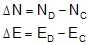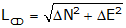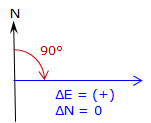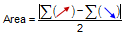1. Forward Computation

A Forward Computation is used to calculate a point's coordinates from distance and direction data from another set of coordinates:Figure C-1 Forward Computation Equation C-1

Eqn (C-1) is a combination of the latitude and departure and coordinate equations from the Traverse Computations topic.

• North Lat is (+), South Lat is (–)
• East Dep is (+), West Dep is (–)

DirAB can be either a bearing or azimuth:

• If a bearing (0° to 90°) is used you must manually determine the correct algebraic sign for the Lat and Dep based on quadrant.
• Using azimuths (0° to 360°) automatically results in correct signs.

Because point B has two unknowns (NB and EB) it must be connected to point A with two measurments,  distance and direction. Having only one or the other isn't sufficient to determine B's coordinates.

2. Inverse Computation

An Inverse Computation determines the distance and direction between two coordinate pairs.Figure C-2 Inverse ComputationEquation C-2Equation C-3Equation C-4

Coordinate differences, Δ’s, are the to point minus the from point.

The algebraic sign on ß and the resulting direction depend on the quadrant of the line.Figure C-3 Quadrants

 Table C-1 Algebraic sign Direction Quadrant ΔN ΔE β Bearing Azimuth NE + + + N β E β SE - + - S |β| E 180°+β SW - - + S β W 180°+β NW + - - N |β| W 360°+β

A negative ß is a counter-clockwise angle.

These should all look familiar as they're the same equations from the Coordinates chapter of the Traverse Computations topic.

When ΔN = 0, Equation C-4 has no solution.Technically division by 0 is undefined, but actually the result of any number divided by 0 is infinity. Remember from the plot of the tangent function that tan(90°) = tan(270°) = infinity. So what does this mean?

In surveying terms when ΔN = 0 the entire line length is ΔE resulting in a due East (+) or West (-) line, Figure C-4.

 ΔE (+) → Az=90° ΔE (-) → Az=270°Figure C-4 When ΔN = 0

3. Area Computation

The area of a non-crossing closed polygon, Figure C-5,Figure C-5 Polygon Areas

can be computed using the coordinates of its vertices:Equation C-5

In surveying terms using North and East coordinates:Equation C-6

An easy way to remember either equation is graphically:

 X & Y E & N List coordinates in order around exterior. Repeat first coordinate pair at end.Cross multiply.Sum cross-productsAdd the cross multiplication sums, divide by two, and take the absolute value to obtain the area:Equation C-7

The absolute value is used because area could be positive or negative depending on traverse configuration, direction around it, and coordinate order (eg, E & N or N & E). Positive or negative, the area magnitude will be correct.

Hits: 7276